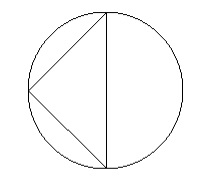# Area of Circumcircle of a Right Angled Triangle?

The area of circumcircle of a right-angle triangle when the hypotenuse(H) of the triangle is given is found using the formula πH2/4.

This formula is derived using the fact that the circumcircle touches all the corners of the triangle, in this case the maximum length between two points in the hypotheses which passes through the center of the circle. This makes the hypotenuse the diameter of the circle.

This is why the area of circle which is πd2/4. (d = 2r) replaces the d by H.## Example

Hypotenuse = 8

Area of circle = 50.26

## Example Code

Live Demo

#include <stdio.h>
int main(void) {
int H = 14;
float pie = 3.14;
float area = (float)((pie*H*H)/4);
printf("the area of circumcircle of a right angled triangle of Hypotenuse %d is %f",H,area);
return 0;
}

## Output

the area of circumcircle of a right angled triangle of Hypotenuse 14 is 153.860016